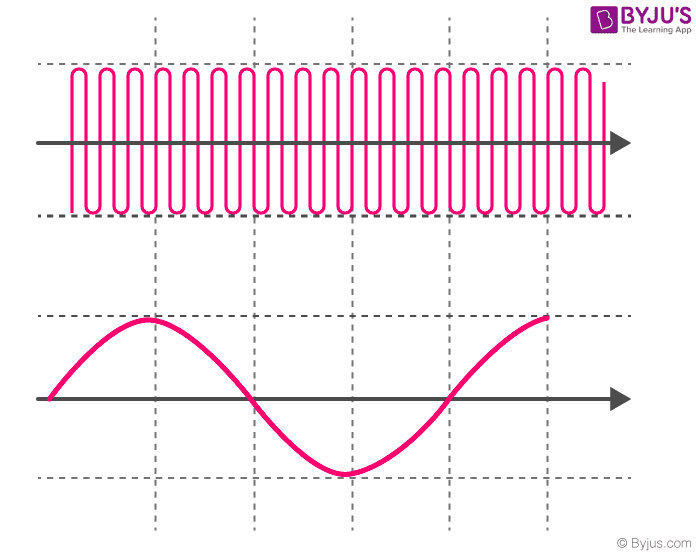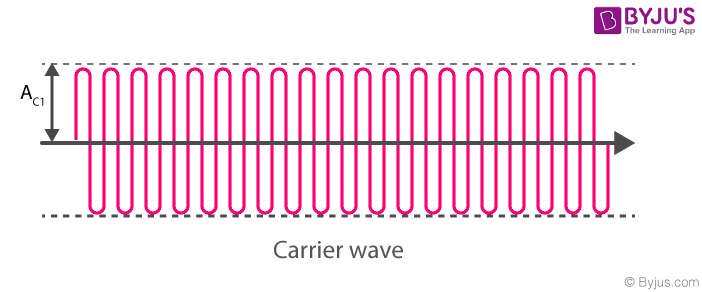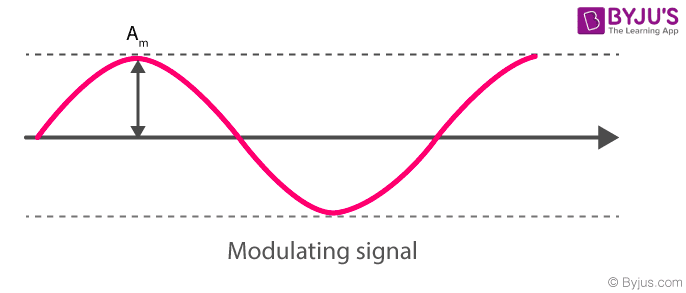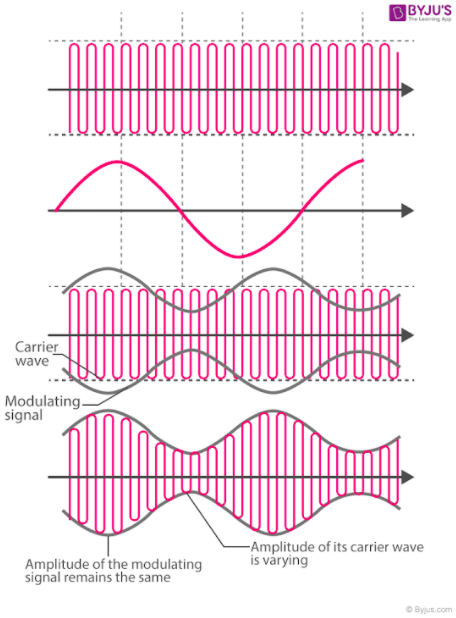Win up to 100% scholarship on Aakash BYJU'S JEE/NEET courses with ABNAT Win up to 100% scholarship on Aakash BYJU'S JEE/NEET courses with ABNAT

# Amplitude Modulation

Amplitude modulation is a process by which the wave signal is transmitted by modulating the amplitude of the signal. It is often called AM and is commonly used in transmitting a piece of information through a radio carrier wave. Amplitude modulation is mostly used in the form of electronic communication.

Currently, this technique is used in many areas of communication, such as in portable two-way radios, citizens band radios, VHF aircraft radios and in modems for computers. Amplitude modulation is also used to refer to mediumwave AM radio broadcasting.

## What Is Amplitude Modulation?

Amplitude modulation, or just AM, is one of the earliest modulation methods that is used in transmitting information over the radio. This technique was devised in the 20th century at a time when  Landell de Moura and Reginald Fessenden were conducting experiments using a radiotelephone in the 1900s. After successful attempts, the modulation technique was established and used in electronic communication.

In general, amplitude modulation definition is given as a type of modulation where the amplitude of the carrier wave varies in some proportion with respect to the modulating data or the signal.

As for the mechanism, when amplitude modulation is used, there is a variation in the amplitude of the carrier. Here, the voltage or the power level of the information signal changes the amplitude of the carrier. In AM, the carrier does not vary in amplitude. However, the modulating data is in the form of signal components consisting of frequencies either higher or lower than that of the carrier. The signal components are known as sidebands, and the sideband power is responsible for the variations in the overall amplitude of the signal.

The AM technique is totally different from frequency modulation and phase modulation, where the frequency of the carrier signal is varied in the first case and in the second one, the phase is varied.

## Types of Amplitude Modulation

There are three main types of amplitude modulation. They are

• Double Sideband-suppressed Carrier Modulation (DSB-SC)
• Single Sideband Modulation (SSB)
• Vestigial Sideband Modulation (VSB)

### Designations by ITU

The International Telecommunication Union (ITU) designated different types of amplitude modulation in 1982. They are as follows:

Designation Description
A3E Double-sideband a full-carrier
R3E Single-sideband reduced-carrier
H3E Single-sideband full-carrier
J3E Single-sideband suppressed-carrier
B8E Independent-sideband emission
C3F Vestigial-sideband

## Communication Systems and Modulation

We are studying modulation under communication systems. They are used to transmit and receive messages (information) from one place to another place in the form of electronic signals, and they are carried out in two different ways.

(i) Analog signal transmission

(ii) Digital signal transmission

So, we can represent an analogue electronic signal (information) as follows:

$$\begin{array}{l}\left.\begin{matrix} m(t)={{A}_{m}}\cos ({{\omega }_{m}}t+\theta ) \\ (or) \\ m(t)={{A}_{m}}\sin ({{\omega }_{m}}t+\theta ) \end{matrix}\right\}…………….1\end{array}$$
.

We can represent the analogue electronic signal either as a sine (or) cosine wave. Every wave will have an amplitude and phase.

Where m(t) = Modulating signal (input signal) or baseband signal

Am = Amplitude of the modulating signal

mt + Ɵ) = Phase of the signal phase contains both frequency (ωmt) ad angle (Ɵ) term

### What Is Modulation?

Basically, it is a process in a communication system. For communication, we need some fundamental elements. One is the high-frequency carrier wave, and the other is the information that has to be transmitted (modulating signal) or input signal. These are essential for communication which is done using a device from one place to another. All in all, we need the help of the communication system.

An electronic communication system converts our message (information) into an electronic signal, and the electronic signal is carried out by carrier waves to the destination.Message (information)

or

Modulating signal

The superposition of modulating signal onto a carrier wave is known as modulation.

Modulation is defined as,

Varying any one of the fundamental parameters of a carrier wave in accordance with the modulating signal. A carrier wave can be represented as a sine or cosine.

C(t) = Ac sin (ωct + Ɵ)

### Amplitude Phase

If we vary the amplitude of the carrier wave in accordance with the modulating signal (input signal), it is known as amplitude modulation.

Similarly, it can be frequency modulation and phase modulation, too. In other words, modulation is the phenomenon of “superimposition of the modulating signal (input signal) into the carrier wave”.

## Why Do We Need Modulation?

Practically speaking, modulation is required for

• High range transmission
• Quality of transmission
• To avoid the overlapping of signals

### High Range Transmission: (Effective Length of Antenna)

For effective communication, the length of the antenna should be λ/4 times the modulating signal.

Hmin = λ/4

λ – Wavelength of the modulating signal or transmitting signal H> λ/4

For example, if I need to transmit a signal of a frequency of f = 20 kHz

As we know, c = f λ

3 × 108 = 20 × 103 (λ)

$$\begin{array}{l}\frac{3\times {{10}^{8}}}{2\times {{10}^{4}}}=\lambda\end{array}$$
$$\begin{array}{l}\lambda =\frac{3}{2}\times {{10}^{4}}\end{array}$$

So

$$\begin{array}{l}{{H}_{\min }}\simeq \frac{\lambda }{4}=\frac{3}{8}\times {{10}^{4}}=0.375\times {{10}^{4}}m\end{array}$$

Hmin = 3750 m

Hmin = 3750 m is practically impossible; for that, we can transmit our modulating signal onto a carrier wave of frequency 1MHz. What we did here is we raised our transmission frequency from 20kHz to 1mHz.

Now, let us find out what the Hmin is needed for good transmission.

c = fλ

3 × 108 = 1×106 (λ)

If we increase the transmitting frequency, wavelengths

$$\begin{array}{l}\frac{3\times {{10}^{8-6}}}{1}=\lambda\end{array}$$
will decreases so, Hmin also decreases.

3 × 102 = λ

$$\begin{array}{l}\Rightarrow f\uparrow and \; \lambda \downarrow \to H\min \downarrow\end{array}$$

λ = 300 m

$$\begin{array}{l}H_{min} = {}^{\lambda }/{}_{4}=\frac{300}{4}=75m\end{array}$$

This is practically possible, so we need modulation to increase the transmission frequency to transmit a low-frequency signal.

### Quality of Transmission: (Power of Transmission by Antenna)

Since, from the Q-factor, we know sharpness or quality is maximum when power is maximum.

Sharpness or quality α power

Power radiated by a linear antenna is

$$\begin{array}{l}P\alpha \frac{1}{{{\lambda }^{2}}}\Rightarrow Power=\frac{\ell }{{{\lambda }^{2}}}\end{array}$$

Where l =  Length of the antenna

λ = Wavelength of the transmitting signal

$$\begin{array}{l}\left.\begin{matrix} for\,small(\lambda ) \\ (or) \\ High\,frequency \end{matrix}\right\} \to \text{Transmission power is high to quality of transmission high}\end{array}$$
.

$$\begin{array}{l}\left.\begin{matrix} for\,high(\lambda ) \\ (or) \\ small\,frequency \end{matrix}\right\} \to \text{Transmission power is small to quality of transmission low}\end{array}$$

### Avoiding the Overlapping of Signals

Two different transmitting stations transmit signals of the same frequency. They will get mixed up or overlap one another. To avoid this, we need to modulate these signals by different carrier waves.

When we talk about amplitude modulation, it is a technique that is used to vary the amplitude of the high-frequency carrier wave in accordance with the amplitude of the modulating signal. But the frequency of the carrier wave remains constant. Now, let us see what carrier waves and modulating signals are.

## Common Terms

Carrier Wave (High Frequency)The amplitude and frequency of a carrier wave remain constant. Generally, it will be high frequency, and it will be a sine or cosine wave of electronic signal; it can be represented as

C(t) = Ac sin wct ……………. 1

Modulating SignalThe modulating signal is nothing but the input signal (electronic signal), which has to be transmitted. It is also a sine or cosine wave; it can be represented as

m(t) = Am sin wmt

Where

Ac and Am = Amplitude of the carrier wave and the modulating signal

sin wct = Phase of the carrier wave

sin wmt = Phase of the modulating signal

## Expression for Amplitude Modulated Wave

We have carrier wave and modulating signals,

$$\begin{array}{l}\left.\begin{matrix} m(t)={{A}_{m}}\,\sin \,{{\omega }_{m}}t\,and \\ \\ c(t)={{A}_{c}}\sin {{\omega }_{c}}t \end{matrix}\right\} \to 1\end{array}$$
.

m(t) = Modulating signal

c(t) = Carrier wave

Am and Ac are the amplitude of modulating signal and carrier wave, respectively, in amplitude modulation. We are superimposing modulating signal into a carrier wave and also varying the amplitude of the carrier wave in accordance with the amplitude of the modulating signal, and the amplitude-modulated wave Cm(t) will be

Cm(t) = (Ac + Am sin ωmt) sin ωct ……………….. 2

This is the general form of an amplitude-modulated wave.

Cm(t) is the amplitude-modulated wave

Where,

A = Ac + Am sin ωmt = Amplitude of the modulated wave

sin wct = Phase of modulated wave

$$\begin{array}{l}{{C}_{m}}(t)={{A}_{c}}\left( 1+\frac{{{A}_{m}}}{{{A}_{c}}}\sin \,{{\omega }_{m}}t \right)\sin {{\omega }_{c}}t\end{array}$$
$$\begin{array}{l}={{A}_{c}}\sin {{\omega }_{c}}t+\frac{{{A}_{m}}}{{{A}_{c}}}{{A}_{c}}\sin {{\omega }_{m}}t\,\sin {{\omega }_{c}}t\end{array}$$

Where,

$$\begin{array}{l}\frac{{{A}_{m}}}{{{A}_{c}}}=\mu = \text{modulation index}\end{array}$$

Cm (t) = Ac sin ωct + Acμ sin ωmt sinωct

We can rewrite the above equation as

$$\begin{array}{l}\sin A\sin B=\frac{1}{2}\left[ \cos (A-B)-\cos (A+B) \right]\end{array}$$
$$\begin{array}{l}={{A}_{c}}\sin {{\omega }_{c}}t+{{A}_{c}}\mu \frac{1}{2}\left[ \cos ({{\omega }_{c}}-{{\omega }_{m}})-\cos \left( {{\omega }_{c}}+{{\omega }_{m}} \right) \right]\end{array}$$
$$\begin{array}{l}{{c}_{m}}(t)={{A}_{c}}\sin {{\omega }_{c}}t+\frac{{{A}_{c}}\mu }{2}\cos ({{\omega }_{c}}-{{\omega }_{m}})-\frac{{{A}_{c}}\mu }{2}\cos \left( {{\omega }_{c}}+{{\omega }_{m}} \right)……\,3\end{array}$$

From equation 3, we can see amplitude modulated wave is the sum of three sine or cosine waves.

### Frequencies of Amplitude Modulated Wave

There are three frequencies in amplitude modulated wave – f1, f2 and f3 – corresponding to ωc, ωc + ωm and ωc – ωm, respectively.

ω1 = ωc → it is corresponding f1 = fc

ω2 = ωc + ωm → it is corresponding f2 = fc + fm

ω3 = ωc – ωm → it is corresponding f3 = fc – fm

Where fc → Carrier wave frequency

fc + fm → Upper side band frequency

fc – fm → Lower side band frequency

fm → Modulating signal frequency

In general, fc > > fm

Bandwidth: (BW) It is the difference between the highest and lowest frequencies of the signal.

BW = Upper sideband frequency – Lower sideband frequency (fc – fm)

Or

BW=fmaxfmin

BW = fc + fm – fc + fm = 2 fm

BW = 2fm = Twice the frequency of the modulating signal

Modulation Index

It is the ratio of the amplitude of the modulating signal to the amplitude of the carrier wave.

$$\begin{array}{l}\mu =\frac{{{A}_{m}}}{{{A}_{c}}}=\frac{\text{Amplitude of modulating signal}}{\text{Amplitude of carrier wave}}\end{array}$$

## Amplitude Modulated Waveform

The waveform representation of amplitude modulated wave is given below.1. Carrier wave

2. Modulating signal

3. Superposition of the carrier wave and modulating signal

4. Amplitude modulated wave

Summary:

Carrier wave, c(t) = Ac sin wct

Modulating single m(t) = Am sin wmt

Amplitude modulate wave (m(t) = (Ac + Am sin ωmt) sin ωct

$$\begin{array}{l}\text{modulation index}(\mu )=\frac{{{A}_{m}}}{{{A}_{c}}}=\frac{\text{Amplitude of modulating signal}}{\text{Amplitude of carrier wave}}\end{array}$$

Frequencies of modulated wave → fc, fc + fm, fc – fm

Bandwidth (w) = fc + fm – (fc – fm) = 2fm

 Advantages Disadvantages Amplitude modulation is easier to implement. When it comes to power usage, it is not efficient. Demodulation can be done using a few components and a circuit. It requires a very high bandwidth that is equivalent to that of the highest audio frequency. The receiver used for AM is very cheap. Noise interference is highly noticeable.

## Applications of Amplitude Modulation

While amplitude modulation use has decreased over the years, it is still present and has several applications in certain transmission areas. We will look at them below.

• Broadcast Transmissions: AM is used in broadcasting transmission over the short, medium and long wavebands. Since AM is easy to demodulate, radio receivers for amplitude modulation are, therefore, easier and cheaper to manufacture.
• Air-band Radio: AM is used in VHF transmissions for many airborne applications, such as ground-to-air radio communications or two-way radio links, for ground staff personnel.
• Single Sideband: Amplitude modulation in this form is used for HF radio links or point-to-point HF links. AM uses a lower bandwidth and provides more effective use of the transmitted power.
• Quadrature Amplitude Modulation: AM is used extensively in transmitting data in several ways, including short-range wireless links, such as Wi-Fi to cellular telecommunications and others.

These are some of the important applications of amplitude modulation.

## Demodulation Methods

The most simple AM demodulator is made up of a diode that acts as an envelope detector. The product detector, which is another type of demodulator, can offer better-quality demodulation but with a complex additional circuit.

## Solved Problems

Question 1:

A carrier wave of frequency f = 1mHz with a pack voltage of 20V is used to modulate a signal of frequency 1kHz with a pack voltage of 10v. Find out the following:

(i) μ

(ii) Frequencies of the modulated wave

(iii) Bandwidth

Solution:

(i)

$$\begin{array}{l}\mu =\frac{{{A}_{m}}}{{{A}_{c}}}=\frac{10v}{20v}=\frac{1}{2}=0.5\end{array}$$

(ii) Frequencies of modulated wave

f → fc, fc + fm and fc – fm

fc = 1mHz, fm = 1kHz

fc + fm = 1×106 + 1×103 = 1001 ×103 = 1001 kHz

fc – fm = 1×106 – 1×103 = 999 × 103 = 999 kHz

(iii) Bandwidth: (W)

(W) = Upper side band frequency – Lower side band frequency

= fc + fm – (fc – fm)

= 2fm = 1001 kHz – 999 kHz = 2 kHz

Question 2:

y = 10 cos (1800 πt) + 20 cos 2000 πt + 10 cos 2200 πt. Find the modulation index (μ) of the given wave.

Solution:

As we know, the expression for amplitude modulated wave is

Cm(t) = (Ac + Am cos ωmt) cos ωct ……………… 1

So, we have to bring the given wave equation into the known form

y = 10 [cos(1800 πt) + cos (2200πt)] + 20 cos 2000 πt

We can rewrite the above equation as

$$\begin{array}{l}\cos A+\cos B=2\cos \left( \frac{A+B}{2} \right)\cos \left( \frac{A-B}{2} \right)\end{array}$$

cos(2000 πt) + cos (1800 πt) = 2 cos 2000 πt cos 200 πt

$$\begin{array}{l}y=10\left[ 2\cos (2000\pi t)\cos (200\pi t) \right]+20\cos 2000\pi t\end{array}$$
$$\begin{array}{l}y=\cos 2000\pi t\left[ 20+20\cos (200\pi t) \right]……2\end{array}$$

Compare equations 1 and 2.

Ac = 20

Am = 20

Then, the modulation index (μ)

$$\begin{array}{l}\mu =\frac{Am}{Ac}=\frac{20}{20}=1\end{array}$$

We can also find the frequencies of the modulated wave and B and width

(ii) Frequencies off the modulated wave

We know frequencies are fc, fc + fm and fc – fm from the modulated wave expression.

$$\begin{array}{l}y=\cos 2000\pi t[20+20\cos (200\pi t)]\,…………\,3\end{array}$$

y = (Ac + Am cos ωmt) cos ωct ……………….. 4

Comparing equations 3 and 4, we get

cos ωmt = cos (200 πt)

ωm = 200 π

2 πfm = 200 π

fm = 100 Hz

Similarly,

cos ωct = cos 2000 πt

ωc = 2000 π

2πfc = 2000 π

fc = 1000 Hz

fc, fc + fm and fc – fm, respectively, 1000 Hz, 1100 Hz and 900 Hz.

(iii) Bandwidth (W)

W = fc + fm – (fc – fm) = 2fm

W = 200 Hz

## NCERT Questions on Amplitude Modulation

1. Why are carrier waves of higher frequency compared to modulating signals?

(i) High-frequency carrier waves effectively reduce the size of the antenna, which increases transmission range.

(ii) They convert wideband signal into a narrowband signal which can easily be recovered at the receiving end.

2. Define modulation index.

The modulation index is defined as the ratio of the amplitude of the modulating signal to the amplitude of the carrier wave (μ).

$$\begin{array}{l}\mu =\frac{{{A}_{m}}}{{{A}_{c}}}\end{array}$$

3. What happens if μ > 1?

As we know, the range of modulation index (μ) should be 0 < μ < 1 if μ > 1. It is said to be over-modulated, and distortion will take place in the modulated signal.

4. Why do we need modulation?

1. To transmit the low-frequency signal to a longer distance.
2. To reduce the length of the antenna.
3. Power radiated by the antenna will be high for high frequency (small wavelength).
4. To avoid the overlapping of modulating signals.

5. Why is the amplitude of the modulating signal kept less than the amplitude of the carrier wave?

To avoid overmodulation. Typically, in overmodulation, the negative half cycle of the modulating signal will be distorted.

## Frequently Asked Questions on Amplitude Modulation

Q1

### Explain Amplitude Modulation.

The amplitude of the wave is altered in proportion to the message signal, such as an audio signal, in amplitude modulation. Amplitude modulation is a modulation technique extensively used in electronic communication to send messages through radio waves.

Q2

### What is the difference between amplitude modulation and frequency modulation?

The distinction is in the modulation or alteration of the carrier wave. To add sound information into amplitude modulation radio, the broadcast’s amplitude, or overall strength, is changed. The carrier signal’s frequency (the number of times per second that the current changes direction) is altered with frequency modulation.

Q3

### Which one is preferable: amplitude modulation or frequency modulation?

Because of the increased bandwidth, FM has better sound quality and is the preferable one.

Test Your Knowledge On Amplitude Modulation!